# M 3: (20 points) Design the stirrup spacing for the beam shown below. Change your design between ...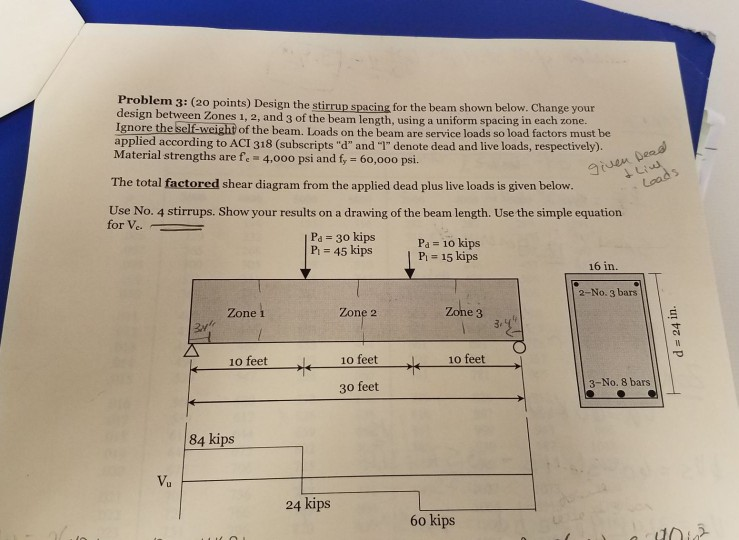m 3: (20 points) Design the stirrup spacing for the beam shown below. Change your design between Zones 1, 2, and 3 of the beam length, using a uniform spacing in each zone. Ignore the self-weight of the beam. Loads on the beam are service loads so load factors must be applied according to ACI 318 (subscripts "d" and T" denote dead and live loads, respectively). Material strengths are fe 4,0oo psi and fy 60,000 psi. The total factored shear diagram from the applied dead plus live loads is given below. Use No. 4 stirrups. Show your results on a drawing of the beam length. Use the simple equation for Ve. Pa 30 kips P 45 kips Pa 10 kips P,-15 kips 16 in. 2-No. 3 bars Zone Zone 2 e 3 寸 10 feet10 feet 30 feet 10feet 3-No. 8 bars 84 kips 24 kips 60 kips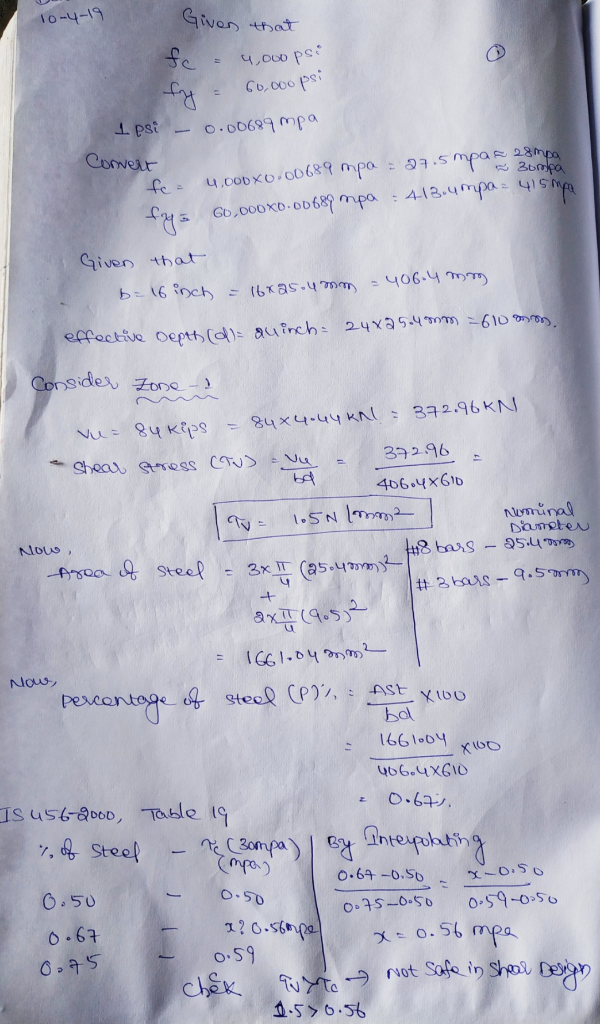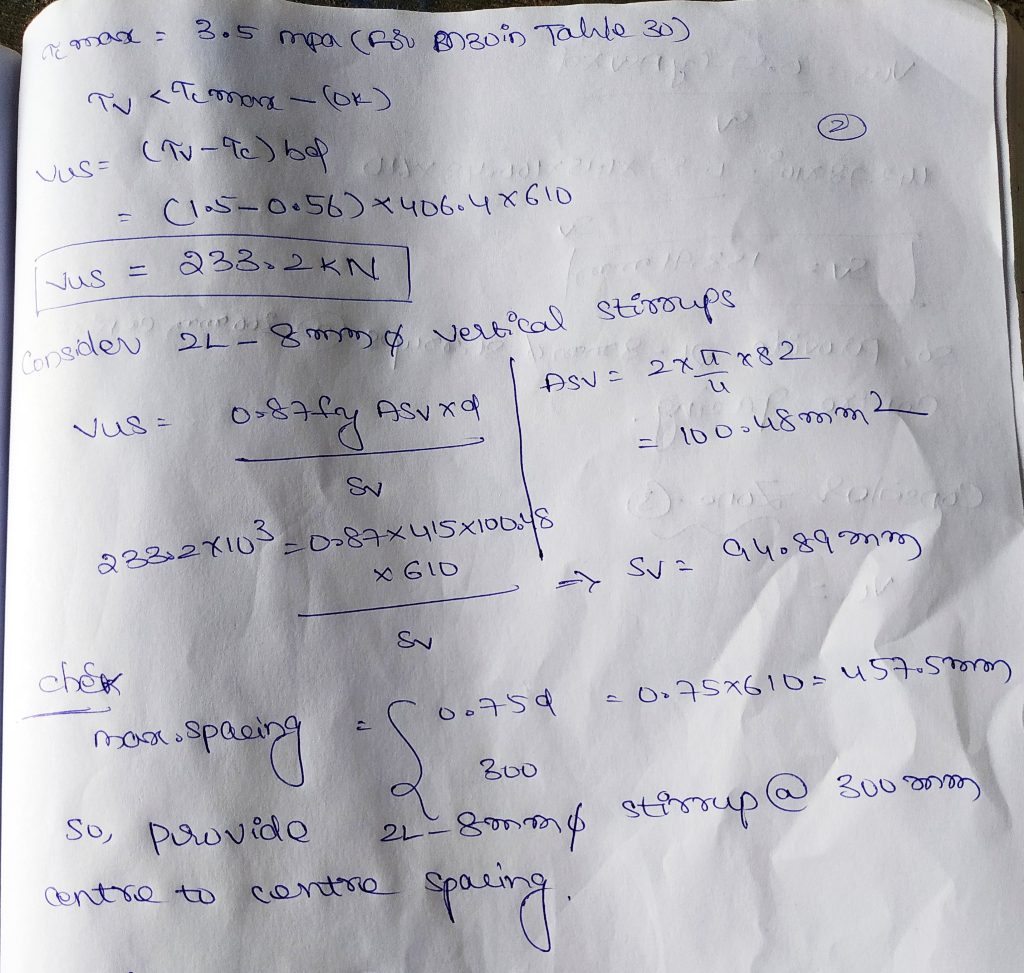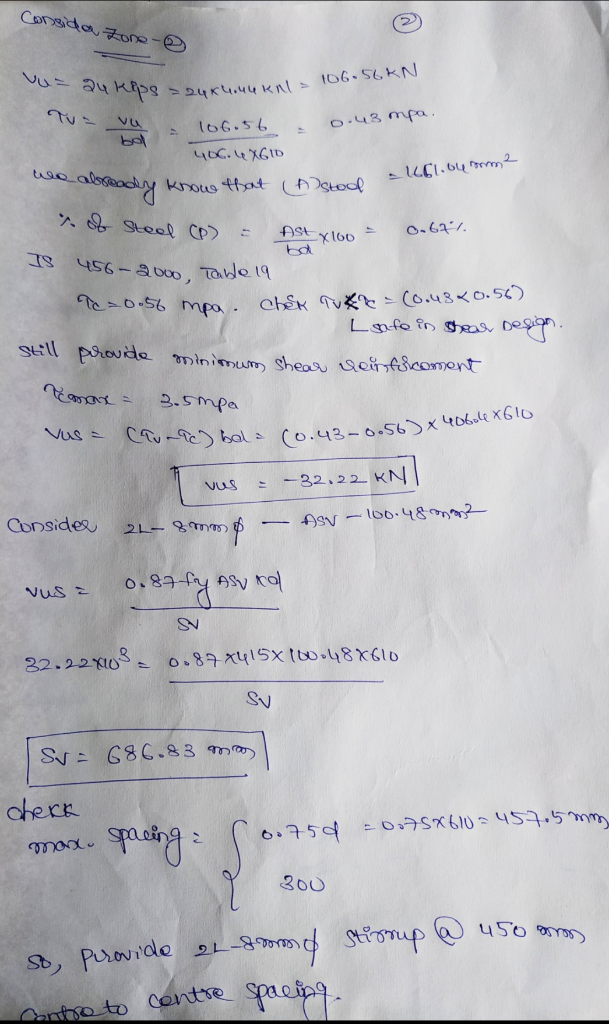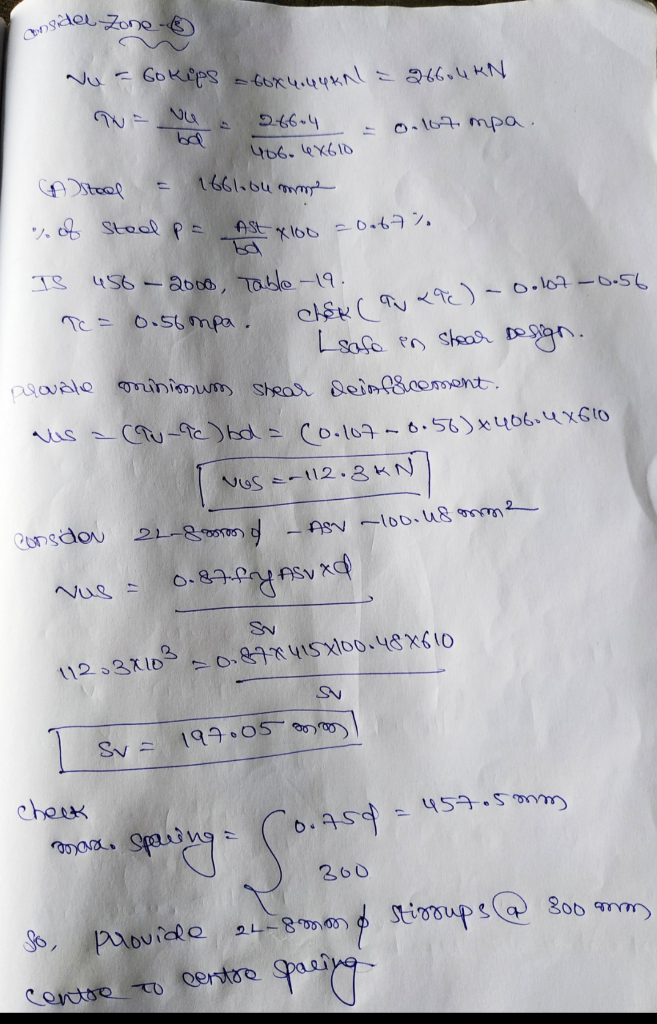##### Add Answer of: M 3: (20 points) Design the stirrup spacing for the beam shown below. Change your design between ...
Similar Homework Help Questions
• ### NOTE Vn(min)= 12.8k Vn(Max)= 81.9k 2) Design the stirrups for the beam using f'4000 psi concrete and # 3 Grade 60 s...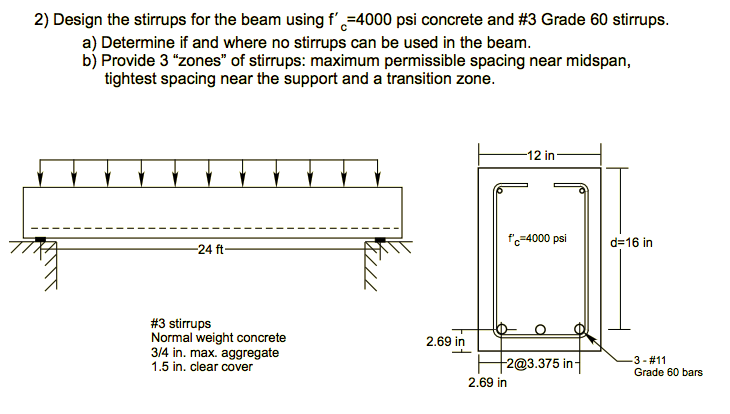NOTE Vn(min)= 12.8k Vn(Max)= 81.9k 2) Design the stirrups for the beam using f'4000 psi concrete and # 3 Grade 60 stirrups. a) Determine if and where no stirrups can be used in the beam. b) Provide 3 "zones" of stirrups: maximum permissible spacing near midspan, tightest spacing near the support and a transition zone 12 in f4000 psi d 16 in 24 ft #3 stirrups Normal weight concrete 3/4 in. max. aggregate 1.5 in. clear cover 2.69 in 3...

• ### Determine if the beam shown in the figure is adequate as governed by the ACI Code (318-11). The l...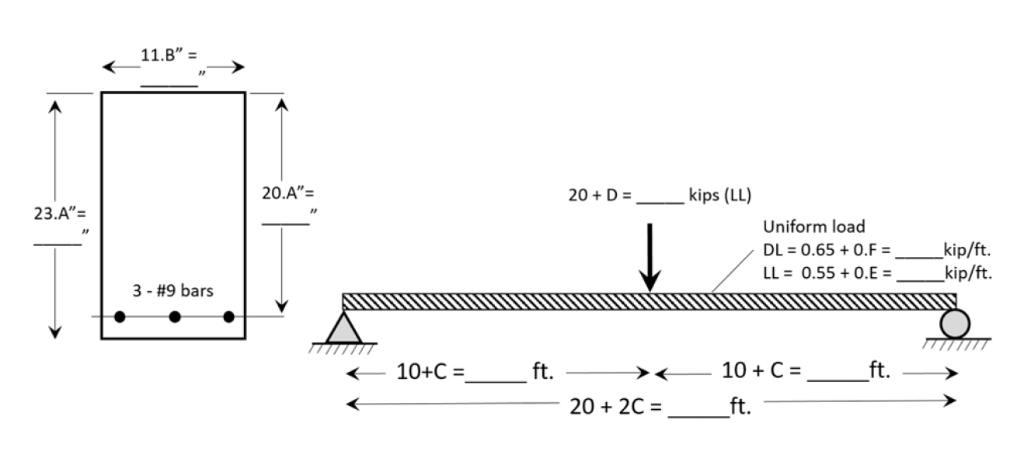Determine if the beam shown in the figure is adequate as governed by the ACI Code (318-11). The loads shown are service loads. The uniformly distributed load is DL = 0.65 + 0.F = _________ kip/ft, LL = 0.55 + 0.E = ________ kip/ft. The dead load excludes the beam weight. The point load is a live load. f’c = 3,000 psi, fy = 40,000 psi. Assume that all variables A,B,C,D,E,F,G are 3.5 kips (LL) 20A"= 20 + D= -...

• ### Design a square tied column to carry axial service loads of 320 kips dead load and 190 kips live load. There is no identified applied moment. Assume that the column is short. Use f’c =4000 psi and fy...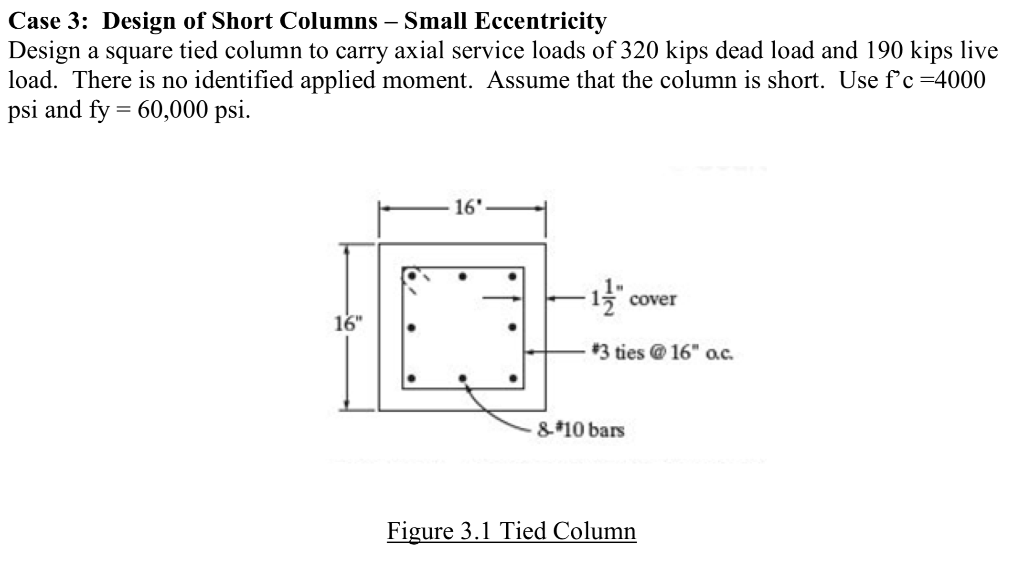Design a square tied column to carry axial service loads of 320 kips dead load and 190 kips live load. There is no identified applied moment. Assume that the column is short. Use f’c =4000 psi and fy = 60,000 psi. Also, draw the flexural and shear reinforcement on a sketch. Case 3: Design of Short Columns - Small Eccentricity Design a square tied column to carry axial service loads of 320 kips dead load and 190 kips live load....

• ### 1. A rectangular beam with b 16 in., d-17 in., and h 20 in. has a clear span of 24 ft. as shown b...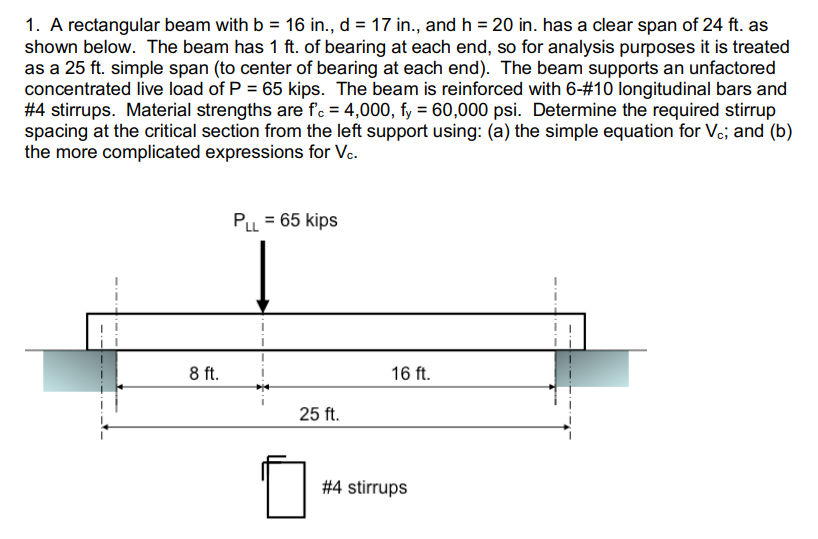1. A rectangular beam with b 16 in., d-17 in., and h 20 in. has a clear span of 24 ft. as shown below. The beam has 1 ft. of bearing at each end, so for analysis purposes it is treated as a 25 ft. simple span (to center of bearing at each end). The beam supports an unfactored concentrated live load of P-65 kips. The beam is reinforced with 6-#10 longitudinal bars and #4 stirrups. Material strengths are fc-4,000,...

• ### Concrete cantilever beam design

Design a rectangular section for a cantilever beam that spans 12 feet out and has a dead weight of 2 kip/ft and a live load of 12 kips at the end of the beam. Assumeconcrete weighs 150 lb/ft^3, fy=60000 psi, f'c=4000psi and roe=(1/2)roe balanced. Roe is the ratio of nonprestressed reinforcement in a section, and roe balanced isthe ratio of tensile reinforcing producing balanced strain condition. Show cross sections, including bar sizes, arrangements, and spacing.

• ### Problem Two: The beam shown below part is for an interior span and is cast monolithically with a one-way slab. The clear span for the beam is 30 feet, and the clear spacing between beams is 10 fee...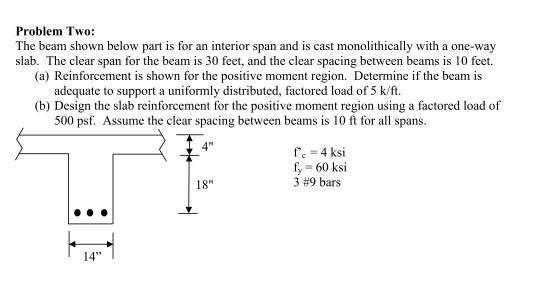Problem Two: The beam shown below part is for an interior span and is cast monolithically with a one-way slab. The clear span for the beam is 30 feet, and the clear spacing between beams is 10 feet. (a) Reinforcement is shown for the positive moment region. Determine if the beam is adequate to support a uniformly distributed, factored load of 5 k/ft. (b) Design the slab reinforcement for the positive moment region using a factored load of 500 psf....

• ### A reinforced concrete beam shown in Figure below is 15-in. wide and has effective depth of31 in. ...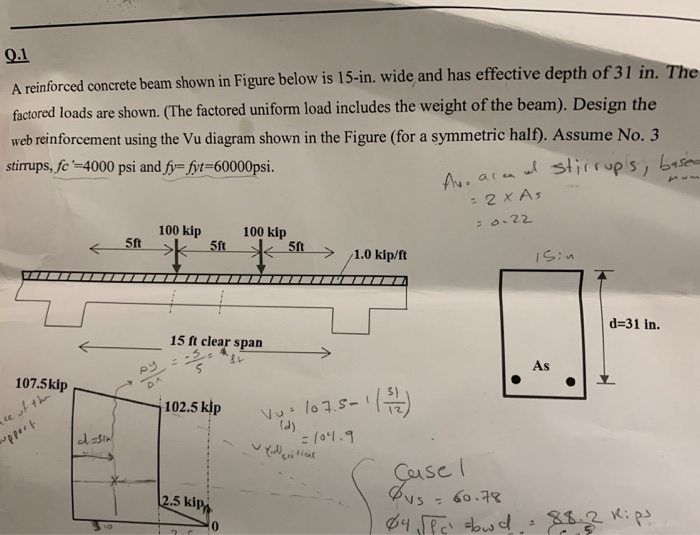A reinforced concrete beam shown in Figure below is 15-in. wide and has effective depth of31 in. The factored loads are shown. (The factored uniform load includes the weight of the beam). Design the web reinforcement using the Vu diagram shown in the Figure (for a symmetric half). Assume No. 3 stirrups,fc '-4000 psi and fy=fyt-60000psi. 100 kip 100 kip d-31 in. 15 ft clear span As s) 102.5 kip se 2.5 ki @y、阪、もw cl.* 3..-K: ps A reinforced concrete...

• ### 3. Figure 3 shows a rigid frame and the factored loads acting on the frame. Design the stirrups in the beam BC only. fe-5000 psi and fy 60000 psi. Hint: Solve for support reactions, draw dd n 50...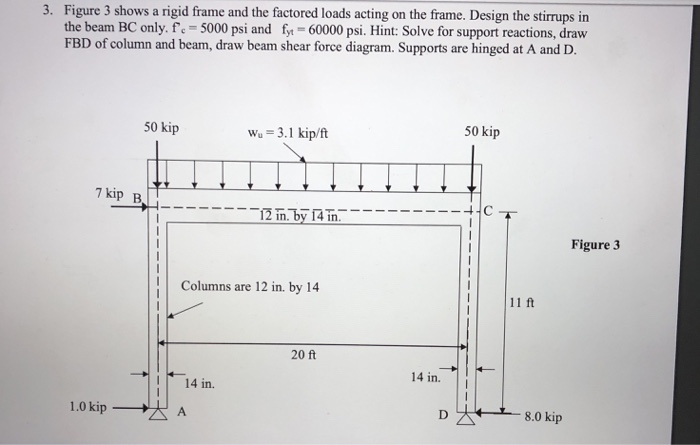3. Figure 3 shows a rigid frame and the factored loads acting on the frame. Design the stirrups in the beam BC only. fe-5000 psi and fy 60000 psi. Hint: Solve for support reactions, draw dd n 50 kip Wu 3.1 kip/ft 50 kip 12 in by A Figure 3 Columns are 12 in. by 14 11 ft 20 ft 14 in. 14 in 1,0 kipーーグ A 3. Figure 3 shows a rigid frame and the factored loads acting on...

• ### 2. A rectangular beam with b = 12 in., d = 17 in., and h = 20 in. spans 23 feet from simple suppo...

2. A rectangular beam with b = 12 in., d = 17 in., and h = 20 in. spans 23 feet from simple supports. Each support is 12 inches wide (clear span between face of supports = 22 feet). The unfactored dead load (excluding self-weight) = 0.9 k/ft, and the unfactored live load = 1.25 k/ft. As = 6.0 in.2 . Material strengths are f’c = 3,500, fy = 60,000 psi. Shear reinforcement consists of #3 stirrups at 8 in....

• ### Need help with E and F please. 3. The beam shown in the figure below is...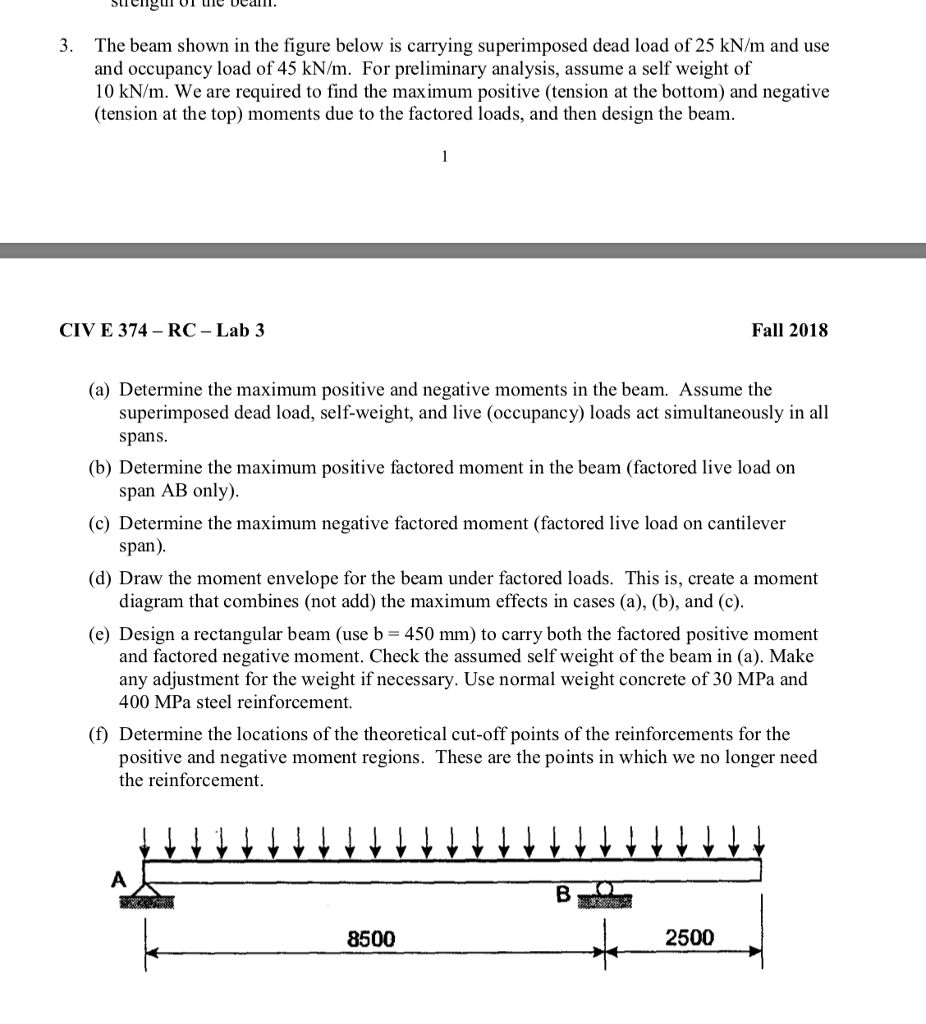Need help with E and F please. 3. The beam shown in the figure below is carrying superimposed dead load of 25 kN/m and use and occupancy load of 45 kN/m. For preliminary analysis, assume a self weight of 10 kN/m. We are required to find the maximum positive (tension at the bottom) and negative (tension at the top) moments due to the factored loads, and then design the beam CIV E 374-RC-Lab 3 Fall 2018 (a) Determine the maximum...

Need Online Homework Help?